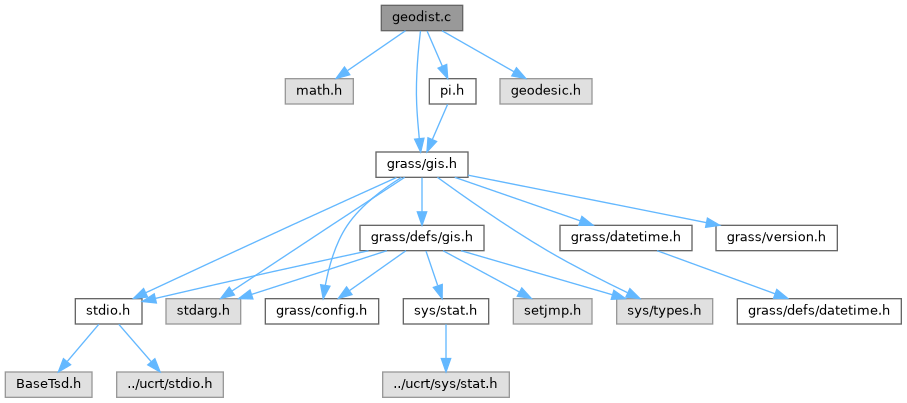GRASS GIS 8 Programmer's Manual  8.2.2dev(2023)-5c00b4e944
geodist.c File Reference

GIS Library - Geodesic distance routines. More...

`#include <math.h>`
`#include <grass/gis.h>`
`#include "pi.h"`
Include dependency graph for geodist.c:Go to the source code of this file.

## Functions

void G_begin_geodesic_distance (double a, double e2)
Begin geodesic distance. More...

void G_set_geodesic_distance_lat1 (double lat1)
Sets geodesic distance lat1. More...

void G_set_geodesic_distance_lat2 (double lat2)
Sets geodesic distance lat2. More...

double G_geodesic_distance_lon_to_lon (double lon1, double lon2)
Calculates geodesic distance. More...

double G_geodesic_distance (double lon1, double lat1, double lon2, double lat2)
Calculates geodesic distance. More...

## Detailed Description

GIS Library - Geodesic distance routines.

Distance from point to point along a geodesic code from Paul D. Thomas, 1970 "Spheroidal Geodesics, Reference Systems, and Local Geometry" U.S. Naval Oceanographic Office, p. 162 Engineering Library 526.3 T36s http://stinet.dtic.mil/oai/oai?&verb=getRecord&metadataPrefix=html&identifier=AD0703541

WARNING: this code is preliminary and may be changed, including calling sequences to any of the functions defined here.

(C) 2001-2009 by the GRASS Development Team

This program is free software under the GNU General Public License (>=v2). Read the file COPYING that comes with GRASS for details.

Definition in file geodist.c.

## ◆ G_begin_geodesic_distance()

 void G_begin_geodesic_distance ( double a, double e2 )

Begin geodesic distance.

Initializes the distance calculations for the ellipsoid with semi-major axis a (in meters) and ellipsoid eccentricity squared e2. It is used only for the latitude-longitude projection.

Note: Must be called once to establish the ellipsoid.

Parameters
 a semi-major axis in meters e2 ellipsoid eccentricity

Definition at line 50 of file geodist.c.

## ◆ G_geodesic_distance()

 double G_geodesic_distance ( double lon1, double lat1, double lon2, double lat2 )

Calculates geodesic distance.

Calculates the geodesic distance from lon1,lat1 to lon2,lat2 in meters.

Note: The calculation of the geodesic distance is fairly costly.

Parameters
 lon1,lat1 longitude,latitude of first point lon2,lat2 longitude,latitude of second point
Returns
distance in meters

Definition at line 196 of file geodist.c.

Referenced by G_distance().

## ◆ G_geodesic_distance_lon_to_lon()

 double G_geodesic_distance_lon_to_lon ( double lon1, double lon2 )

Calculates geodesic distance.

Calculates the geodesic distance from lon1,lat1 to lon2,lat2 in meters where lat1 was the latitude passed to G_set_geodesic_distance_latl() and lat2 was the latitude passed to G_set_geodesic_distance_lat2().

Parameters
 lon1 first longitude lon2 second longitude
Returns
double distance in meters

Definition at line 121 of file geodist.c.

Referenced by G_geodesic_distance().

## ◆ G_set_geodesic_distance_lat1()

 void G_set_geodesic_distance_lat1 ( double lat1 )

Sets geodesic distance lat1.

Set the first latitude.

Note: Must be called first.

Parameters
 lat1 first latitude
Returns

Definition at line 69 of file geodist.c.

Referenced by G_geodesic_distance().

## ◆ G_set_geodesic_distance_lat2()

 void G_set_geodesic_distance_lat2 ( double lat2 )

Sets geodesic distance lat2.

Set the second latitude.

Note: Must be called second.

Parameters
 lat2 second latitidue

Definition at line 83 of file geodist.c.